# 12-240/Homework Assignment 1

(diff) ← Older revision | Latest revision (diff) | Newer revision → (diff)
1. Suppose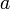$a$ and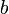$b$ are nonzero elements of a field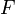$F$. Using only the field axioms, prove that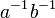$a^{-1}b^{-1}$ is a multiplicative inverse of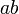$ab$. State which axioms are used in your proof.
2. Prove that if$a$ and$b$ are elements of a field$F$, then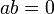$ab=0$ if and only if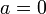$a=0$ or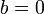$b=0$.
3. Write the following complex numbers in the form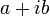$a+ib$, with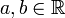$a,b\in{\mathbb R}$:
1.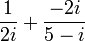$\frac{1}{2i}+\frac{-2i}{5-i}$.
2.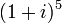$(1+i)^5$.
1. Prove that the set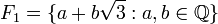$F_1=\{a+b\sqrt{3}:a,b\in{\mathbb Q}\}$ (endowed with the addition and multiplication inherited from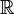${\mathbb R}$) is a field.
2. Is the set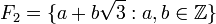$F_2=\{a+b\sqrt{3}:a,b\in{\mathbb Z}\}$ (with the same addition and multiplication) also a field?
4. Let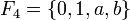$F_4=\{0,1,a,b\}$ be a field containing 4 elements. Assume that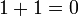$1+1=0$. Prove that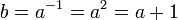$b=a^{-1}=a^2=a+1$. (Hint: For example, for the first equality, show that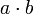$a\cdot b$ cannot equal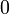$0$,$a$, or$b$.)